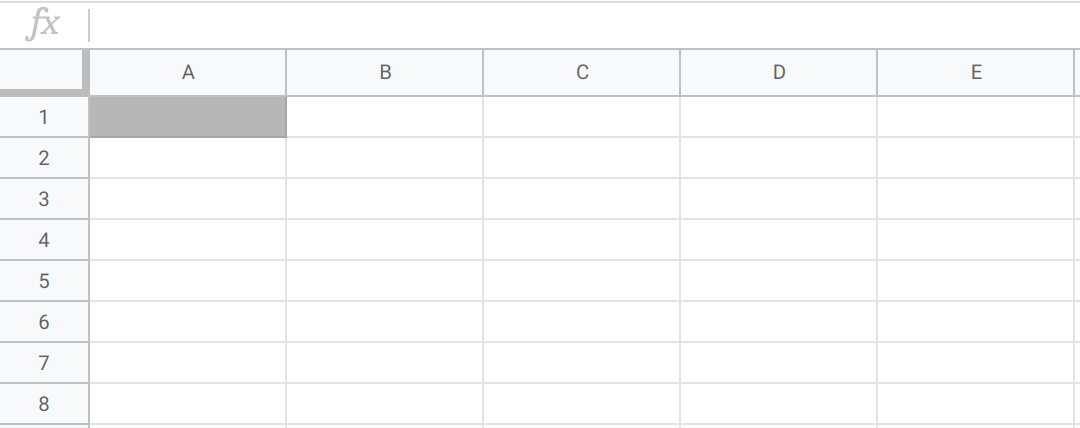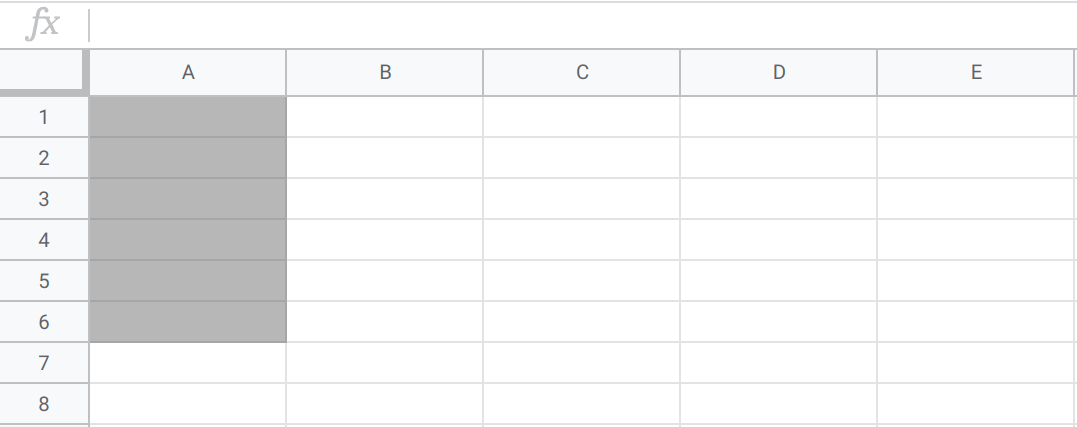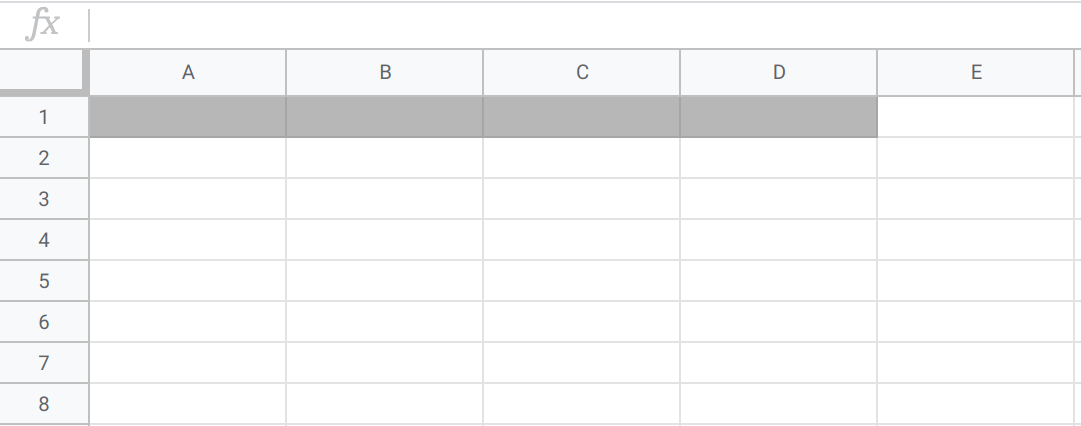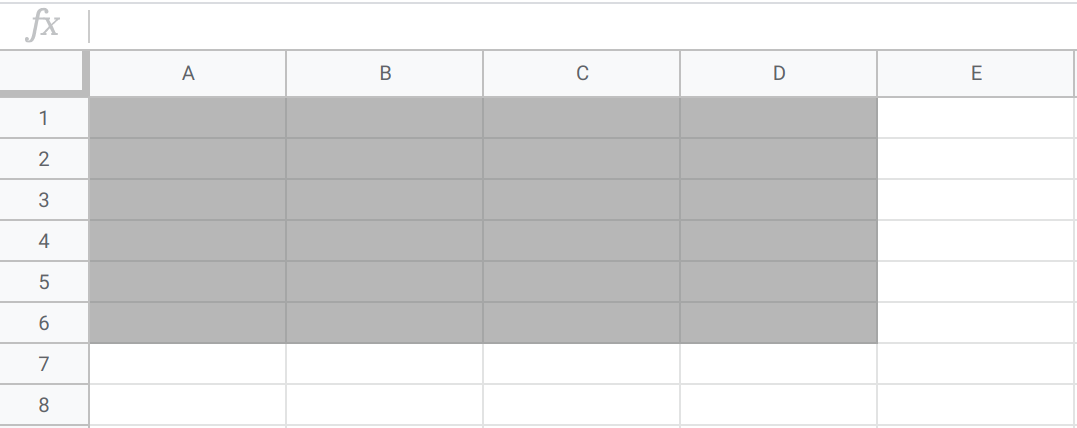# Count and Sum¶

Two of the most basic but most used statistics are the count and sum of a dataset.

Count Definitions

The count of a dataset is the number of observations in the dataset. This applies to all variables.

Sum Definition

The sum of a dataset is the addition of all values in the dataset. This applies only to quantitative variables.

## Applications and Examples¶

The reason that the count is so important is that it can be an indication of how useful the dataset is. For example, suppose you’re trying to find the average height of students at your college of 800 people, so you conduct a survey collecting students’ heights. If your survey contains only 2 heights, then using these results to calculate an average won’t be very useful, since this summary statistic is only representative of 2 students. If, however, your survey contains 600 heights, calculating an average from these results will be more reliable, since this statistic represents 600 of the 800 students.

As an example of using count and sum, suppose you are throwing a homework party. You ask your friends to RSVP if they are attending and to submit how many pizza slices they would like to eat, through a survey. Knowing how many people would be attending will be helpful to you when you purchase snacks, and you can use the count to calculate the number of guests. You could also take the sum of the number of pizza slices your friends want in order to get a better idea of how many pizzas to order.

Calculating these statistics in Sheets is fairly straightforward. For these and other statistics, you’ll need to know cell range notation. To refer to a range of cells in Sheets, type the first cell in the range and the last cell in the range, separated by a colon. For example:

• “A1” refers to cell A1• “A1:A6” means all cells in the A column from rows 1 through 6• “A1:D1” means all cells in the 1 row from columns A through D• “A1:D6” means all cells in rows 1 through 6 and columns A through DCount and Sum Sheets Syntax

• The `COUNT` function returns the number of non-empty cells in a range. The syntax is `=COUNT(cell range)`.

• The `SUM` function returns the addition of a cell range. The syntax is `=SUM(cell range)`.

First, use the `COUNT` function to verify that there are five rows in this dataset, one for each person on the plan.
Now, use the `SUM` function to add up all the values.